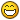# Help with Matrix algorithm

## Recommended Posts

I need some help to optimize algorithm.

I have a matrix of sprites and I am highlighting a part of it. It can be rectangle or square it doesn't matter. I need to hide all sprites of this matrix that do not belong to the given area.

I have this function which is highly non-optimized i.e 4 nested "for" cycles:

``````hideMatrixArea: function(slotTwoDimArray, row, col, numRows, numCols) {
var i = 0,
j = 0,
k = 0,
t = 0,
matrixWidth = slotTwoDimArray.length,
matrixHeigth = slotTwoDimArray.length;

for (i = 0; i < matrixWidth; i += 1, k += 1) {
for (j = 0; j < matrixHeigth; j += 1, t += 1) {
for (k = row; k < row + numRows; k += 1) {
for (t = col; t < col + numCols; t += 1) {
if (!(k === i && t === j)) {
console.log("hello");
this.hideSlot(slotTwoDimArray, i, j);
}
}
}
}
}

return slotTwoDimArray;
},``````

It prints "hello" 15600 times which is a lot.How can I hide slots that are not in the space (row, col) -> (row + numRows, col + numCols) i.e the inverse without using nested "for" loops?

Any ideas?

##### Share on other sites

I'm not entirely clear what you're trying to do... but one data structure you might check out is a spatial hash. You partition two-dimensional space into a series of boxes and, as things move, update which boxes they overlap. When it comes time to check to see what is overlapping what, you can check only the boxes you care about instead of iterating over every object in the game.

Would something like that help?

##### Share on other sites

Assuming you are trying to create a 2d array (matrix) then you need to follw these steps,

1. We declare it as a variable.

``````var grid_9x9 = [
[0, 0, 0, 0, 0, 0, 0, 0, 0],
[0, 0, 0, 0, 1, 1, 1, 1, 0],
[0, 0, 0, 1, 1, 1, 1, 1, 0],
[0, 0, 1, 1, 1, 1, 1, 1, 0],
[0, 1, 1, 1, 1, 1, 1, 1, 0],
[0, 1, 1, 1, 1, 1, 1, 0, 0],
[0, 1, 1, 1, 1, 1, 0, 0, 0],
[0, 1, 1, 1, 1, 0, 0, 0, 0],
[0, 0, 0, 0, 0, 0, 0, 0, 0],
];``````

2. We create another function that can display or array. This function should take in an array as parameter.

``````function Render2dGrid(arr){
for(var row = 0; row < arr.length; row++){
for(var col = 0; col < arr[row].length; col++){
if (arr[row][col] != 0){
//value is 1, render the cell
}else{
//value is 0 do not render cell
}
}
}
}``````

3. We need a function to set the values inside a Rectangle to 0 ( 1 is visible, 0 is not visible). O(x,y) will be the start value and E(x,y) will be the end value of the rectangle selection.

``````function Toggle2dGrid(arr, ox, oy, ex, ey){
var temp;

//we normalize our Origin and End values
if ox > ex then {
temp = ox;
ox = ex;
ex = temp;
}

if oy > ey then {
temp = oy;
oy = ex;
ex = temp;
}

//the if statement checks that our endpoints are not values outside out array range.
if ((ox != ex) && (oy != ey) && (ey <= arr.length) && (ex <= arr[row].length) && (ox => 0) && (oy => 0)){
for(var row = oy; row < ey; row++){
for(var col = ox; col < ex; col++){
//set value to 0
arr[row][col] = 0;
}
}
else {
//Error selection
console.log('Error selection');
}
}``````

In actual use, you first Initiate it, then render it, then toggle, then render.